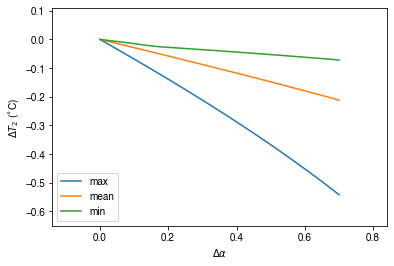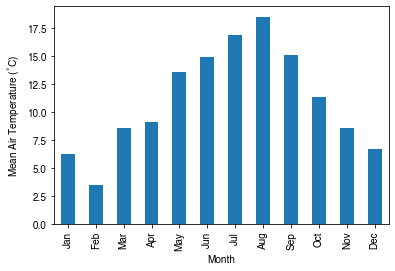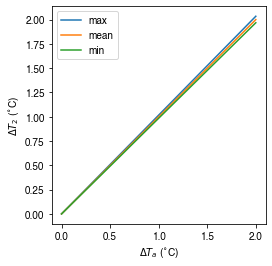# Impact Studies Using SuPy¶

## Aim¶

In this tutorial, we aim to perform sensitivity analysis using supy in a parallel mode to investigate the impacts on urban climate of

1. surface properties: the physical attributes of land covers (e.g., albedo, water holding capacity, etc.)

2. background climate: longterm meteorological conditions (e.g., air temperature, precipitation, etc.)

### load supy and sample dataset¶

:

from dask import dataframe as dd
import supy as sp

import pandas as pd
import numpy as np

from time import time


:

# load sample datasets

# by default, two years of forcing data are included;
# to save running time for demonstration, we only use one year in this demo
df_forcing=df_forcing.loc['2012'].iloc[1:]

# perform an example run to get output samples for later use
df_output, df_state_final = sp.run_supy(df_forcing, df_state_init)


2020-07-06 00:35:30,550 - SuPy - INFO - All cache cleared.
2020-07-06 00:35:33,162 - SuPy - INFO - ====================
2020-07-06 00:35:33,163 - SuPy - INFO - Simulation period:
2020-07-06 00:35:33,164 - SuPy - INFO -   Start: 2012-01-01 00:05:00
2020-07-06 00:35:33,164 - SuPy - INFO -   End: 2012-12-31 23:55:00
2020-07-06 00:35:33,165 - SuPy - INFO -
2020-07-06 00:35:33,166 - SuPy - INFO - No. of grids: 1
2020-07-06 00:35:33,166 - SuPy - INFO - SuPy is running in serial mode
2020-07-06 00:35:46,945 - SuPy - INFO - Execution time: 13.8 s
2020-07-06 00:35:46,946 - SuPy - INFO - ====================



## Surface properties: surface albedo¶

### Examine the default albedo values loaded from the sample dataset¶

:

df_state_init.alb

:

ind_dim (0,) (1,) (2,) (3,) (4,) (5,) (6,)
grid
1 0.1 0.12 0.1 0.18 0.21 0.18 0.1

### Copy the initial condition DataFrame to have a clean slate for our study¶

Note: DataFrame.copy() defaults to deepcopy

:

df_state_init_test = df_state_init.copy()



### Set the Bldg land cover to 100% for this study¶

:

df_state_init_test.sfr = 0
df_state_init_test.loc[:, ('sfr', '(1,)')] = 1
df_state_init_test.sfr


:

ind_dim (0,) (1,) (2,) (3,) (4,) (5,) (6,)
grid
1 0 1 0 0 0 0 0

### Construct a df_state_init_x dataframe to perform supy simulations with specified albedo¶

:

# create a df_state_init_x with different surface properties
n_test = 48
list_alb_test = np.linspace(0.1, 0.8, n_test).round(2)
df_state_init_x = df_state_init_test.append(
[df_state_init_test]*(n_test-1), ignore_index=True)

# here we modify surface albedo
df_state_init_x.loc[:, ('alb', '(1,)')] = list_alb_test
df_state_init_x.index=df_state_init_x.index.rename('grid')



### Conduct simulations with supy¶

:

df_forcing_part = df_forcing.loc['2012 01':'2012 07']
df_res_alb_test,df_state_final_x = sp.run_supy(df_forcing_part, df_state_init_x,logging_level=90)


### Examine the simulation results¶

:

# choose results of July 2012 for analysis
df_res_alb_test_july = df_res_alb_test.SUEWS.unstack(0).loc["2012 7"]
df_res_alb_T2_stat = df_res_alb_test_july.T2.describe()
df_res_alb_T2_diff = df_res_alb_T2_stat.transform(
lambda x: x - df_res_alb_T2_stat.iloc[:, 0]
)
df_res_alb_T2_diff.columns = list_alb_test - list_alb_test

:

ax_temp_diff = df_res_alb_T2_diff.loc[["max", "mean", "min"]].T.plot()
_ = ax_temp_diff.set_ylabel("$\Delta T_2$ ($^{\circ}}$C)")
_ = ax_temp_diff.set_xlabel(r"$\Delta\alpha$")
ax_temp_diff.margins(x=0.2, y=0.2)## Background climate: air temperature¶

### Examine the monthly climatology of air temperature loaded from the sample dataset¶

:

df_plot = df_forcing.Tair.loc["2012"].resample("1m").mean()
ax_temp = df_plot.plot.bar(color="tab:blue")
_ = ax_temp.set_xticklabels(df_plot.index.strftime("%b"))
_ = ax_temp.set_ylabel("Mean Air Temperature ($^\degree$C)")
_ = ax_temp.set_xlabel("Month")### Construct a function to perform parallel supy simulations with specified diff_airtemp_test: the difference in air temperature between the one used in simulation and loaded from sample dataset.¶

Note

forcing data df_forcing has different data structure from df_state_init; so we need to modify run_supy_mgrids to implement a run_supy_mclims for different climate scenarios*

Let’s start the implementation of run_supy_mclims with a small problem of four forcing groups (i.e., climate scenarios), where the air temperatures differ from the baseline scenario with a constant bias.

:

# save loaded sample datasets
df_forcing_part_test = df_forcing.loc['2012 1':'2012 7'].copy()
df_state_init_test = df_state_init.copy()

:

from dask import delayed
# create a dict with four forcing conditions as a test
n_test = 4
list_TairDiff_test = np.linspace(0., 2, n_test).round(2)
dict_df_forcing_x = {
tairdiff: df_forcing_part_test.copy()
for tairdiff in list_TairDiff_test}
for tairdiff in dict_df_forcing_x:
dict_df_forcing_x[tairdiff].loc[:, 'Tair'] += tairdiff

dd_forcing_x = {
k: delayed(sp.run_supy)(df, df_state_init_test,logging_level=90)
for k, df in dict_df_forcing_x.items()}

df_res_tairdiff_test0 = delayed(pd.concat)(
dd_forcing_x,
keys=list_TairDiff_test,
names=['tairdiff'],
)

:

# test the performance of a parallel run
t0 = time()
df_res_tairdiff_test = df_res_tairdiff_test0\
.reset_index('grid', drop=True)
t1 = time()
t_par = t1 - t0
print(f'Execution time: {t_par:.2f} s')

Execution time: 29.80 s

:

# function for multi-climate run_supy
# wrapping the above code into one
def run_supy_mclims(df_state_init, dict_df_forcing_mclims):
dd_forcing_x = {
k: delayed(sp.run_supy)(df, df_state_init_test,logging_level=90)
for k, df in dict_df_forcing_x.items()}
df_output_mclims0 = delayed(pd.concat)(
dd_forcing_x,
keys=list(dict_df_forcing_x.keys()),
names=['clm'],
df_output_mclims = df_output_mclims0.reset_index('grid', drop=True)

return df_output_mclims


### Construct dict_df_forcing_x with multiple forcing DataFrames¶

:

# save loaded sample datasets
df_forcing_part_test = df_forcing.loc['2012 1':'2012 7'].copy()
df_state_init_test = df_state_init.copy()

# create a dict with a number of forcing conditions
n_test = 12 # can be set with a smaller value to save simulation time
list_TairDiff_test = np.linspace(0., 2, n_test).round(2)
dict_df_forcing_x = {
tairdiff: df_forcing_part_test.copy()
for tairdiff in list_TairDiff_test}
for tairdiff in dict_df_forcing_x:
dict_df_forcing_x[tairdiff].loc[:, 'Tair'] += tairdiff


### Perform simulations¶

:

# run parallel simulations using run_supy_mclims
t0 = time()
df_airtemp_test_x = run_supy_mclims(df_state_init_test, dict_df_forcing_x)
t1 = time()
t_par = t1-t0
print(f'Execution time: {t_par:.2f} s')

Execution time: 183.60 s


### Examine the results¶

:

df_airtemp_test = df_airtemp_test_x.SUEWS.unstack(0)
df_temp_diff = df_airtemp_test.T2.transform(lambda x: x - df_airtemp_test.T2[0.0])
df_temp_diff_ana = df_temp_diff.loc["2012 7"]
df_temp_diff_stat = df_temp_diff_ana.describe().loc[["max", "mean", "min"]].T

:

ax_temp_diff_stat=df_temp_diff_stat.plot()
_=ax_temp_diff_stat.set_ylabel('$\\Delta T_2$ ($^{\\circ}}$C)')
_=ax_temp_diff_stat.set_xlabel('$\\Delta T_{a}$ ($^{\\circ}}$C)')
ax_temp_diff_stat.set_aspect('equal')The $$T_{2}$$ results indicate the increased $$T_{a}$$ has different impacts on the $$T_{2}$$ metrics (minimum, mean and maximum) but all increase linearly with $$T_{a}.$$ The maximum $$T_{2}$$ has the stronger response compared to the other metrics.